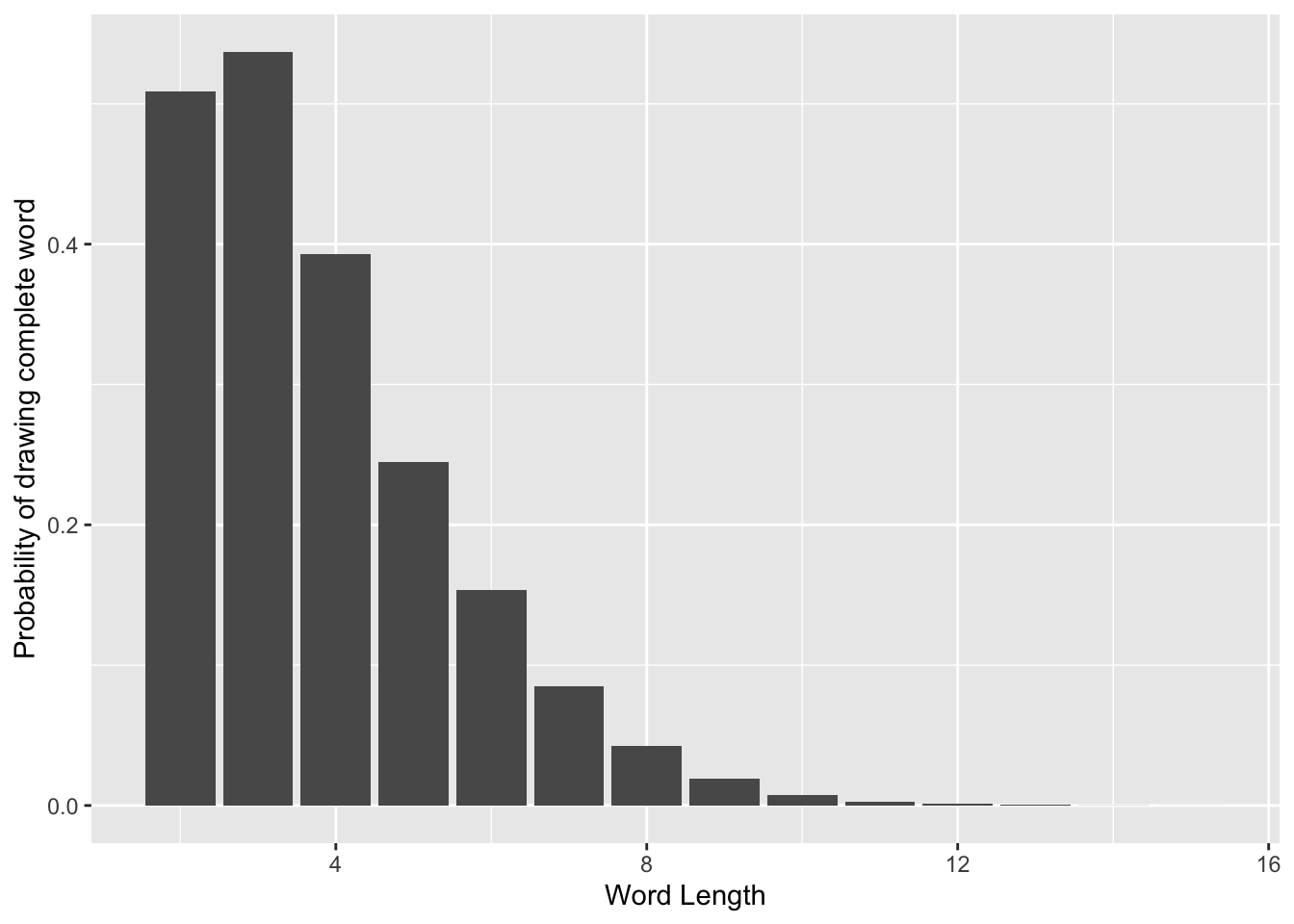Bananagrams Probabilities

There was a fabled game of Bananagrams in which my Dad drew his initial 11 tiles, and immediately spelled:

RASTAFARIAN

If you haven’t played the game, it’s like a free-form version of Scrabble. You start by drawing a number of tiles (typically 11 or 21), and try to form a word or words out of them.

The story made me wonder how likely it is to spell an 11 letter word on the first draw.

The first step is to calculate the probability of drawing a particular word. Consider a bananagrams bag filled with only two letters, S for success and F for failure. Start pulling out tiles from the bag at random, without replacing each tile back in the bag after drawing it, and count how many S tiles you get. The hypergeometric distribution models the probability that you will get a certain number of S tiles for a given number of draws. The multivariate hypergeometric distribution extends this to the multivariate case; that is, it models the probability you’ll draw a certain number of As, Bs, Cs, etc. after drawing a number of tiles from the bag.

Fortunately, the R package extraDistr provides an R version of the multivariate hypergeometric probability mass function. Here’s a function that, given a word of length $$N$$ and the number of each letter tile in a bag, gives the probability of drawing that word in $$N$$ draws:

Using this function, we can find the probability of drawing
RASTAFARIAN:

And the result is $$4.28\times10^{-6}\%$$. Pretty lucky!

Now, what is the probability of drawing any valid 11 letter word to start the game? Note that in most cases, spelling a word using all your 11 tiles excludes the possibility of spelling another word. This suggests the the probability of spelling word $$A$$ OR word $$B$$ is given by $$P(A \cap B)=P(A) + P(B)$$.

However, there is a special case: what if word $$A$$ and word $$B$$ are spelled with the same letters? In order to avoid double counting, we need to only want to include words with the same letters once.

I downloaded a list of words in the SOWPODS scrabble dictionary from a GitHub repository and loaded them into R. To deduplicate words with the same letters, I sorted the letters in each word and removed duplicates:

I then used the word_probability function to calculate the probability of drawing each 11 letter word, and then summed them all up:

Which computes the probability of drawing a valid 11 letter word in the opening tiles to be $$~0.28\%$$.

Now, suppose you start the game by drawing a different number of tiles. We can compute the probability of starting with a valid word for a range of starting tile numbers:Drawing 3 letters has the highest probability of forming a word, at $$53.7\%$$. This validates my strategy of dumping early in the game to get new tiles when I get stuck, because the new letters often help me get out of the rut.